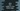# Java program to find the sublist in a list within range### Java program to find sublist in a list :

In this tutorial, we will learn how to find one sublist of a list within a range. The user will enter the starting index and end index of the list. We will print out the sublist using this starting and ending index. Let’s take a look at the Java program first :

### Java program to find sublist in a list :

``````import java.util.*;

public class Main {

public static void main(String[] args) {
//1
Scanner scanner = new Scanner(System.in);

//2
ArrayList numberList = new ArrayList();

//3
for (int i = 0; i <= 100; i++) {
numberList.add(i);
}

//4
System.out.println("Enter starting index between 0 and 101 : ");
int start = scanner.nextInt();

//5
System.out.println("Enter second index between 0 and 101 : ");
int end = scanner.nextInt();

//6
List subList = numberList.subList(start,end);

//7
System.out.println("Sublist : "+subList.toString());

}

}``````

### Explanation :

The commented numbers in the above program denote the step number below :

1. Create one Scanner object to get the inputs from the user.
2. Create one ArrayList.
3. Using one for loop, add elements from 0 to 100 to this arraylist. So, on position i, the value is i for i = 0…100.
4. Ask the user to enter starting index for the sublist. Save it to the variable start.
5. Ask the user to enter ending index for the sublist. Save it to the variable end.
6. Create one sublist by using the subList(startIndex,endIndex) function. Starting index is start and ending index is end.
7. Print out the sublist to the user.

### Sample Output :

``````1
Enter second index between 0 and 101 :
14
Sublist : [1, 2, 3, 4, 5, 6, 7, 8, 9, 10, 11, 12, 13]

Enter starting index between 0 and 101 :
1
Enter second index between 0 and 101 :
13
Sublist : [1, 2, 3, 4, 5, 6, 7, 8, 9, 10, 11, 12]``````